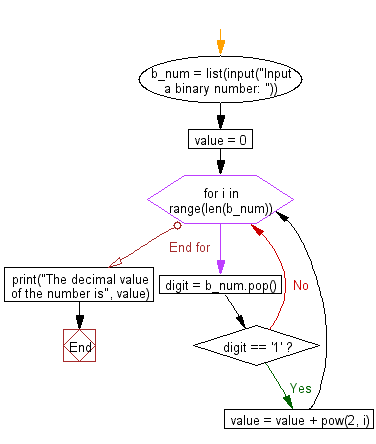﻿ Python Math: Convert a binary number to decimal number - w3resource# Python Math: Convert a binary number to decimal number

## Python Math: Exercise-31 with Solution

Write a Python program to convert a binary number to decimal number.

Sample Solution:-

Python Code:

``````b_num = list(input("Input a binary number: "))
value = 0

for i in range(len(b_num)):
digit = b_num.pop()
if digit == '1':
value = value + pow(2, i)
print("The decimal value of the number is", value)
```
```

Sample Output:

```Input a binary number: 1000001
The decimal value of the number is 65
```

Pictorial Presentation:Flowchart:## Visualize Python code execution:

The following tool visualize what the computer is doing step-by-step as it executes the said program:

Python Code Editor:

Have another way to solve this solution? Contribute your code (and comments) through Disqus.

What is the difficulty level of this exercise?

Test your Programming skills with w3resource's quiz.

﻿

```>>> students = [{'name': 'John', 'score': 98}, {'name': 'Mike', 'score': 94}, {'name': 'Jennifer', 'score': 99}]